# sql: sum over partition by用法

0、select * from wmg_test; —测试数据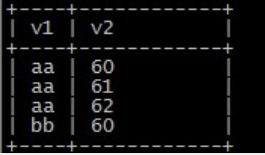1、select v1,v2,sum(v2) over(order by v2) as sum from wmg_test;
–按照 v2排序，累计n+n-1+…+1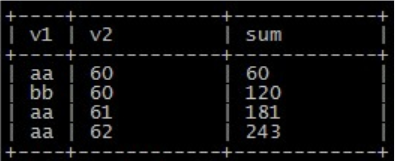2、select v1,v2,sum(v2) over(partition by v1 order by v2) as sum from wmg_test; --先分组，组内在进行 1 中的操作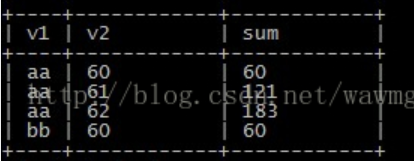3、select v1,v2,sum(v2) over(partition by v1 order by v1) as sum from wmg_test; —稳定排序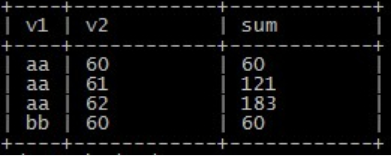4、select v1,v2,sum(v2) over(partition by v1) as sum from wmg_test; --相同key的进行回填处理，没有order by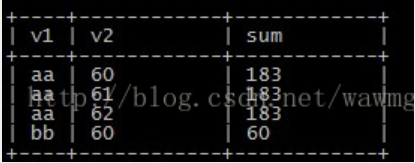5、select distinct v1,sum_01 from (select v1,sum(v2) over(partition by v1) as sum_01 from wmg_test) a;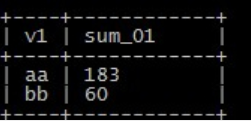6、当然也可以逆序累加，只需order by desc 即可

group 单纯分组
partition 也能分组，但还具备累计的功能
7、max … partition by

Select Pty_Id, Data_Dt,Fin_In, Max(Fin_In) Over(Partition By Pty_Id Order By Data_Dt) As Max_Cost from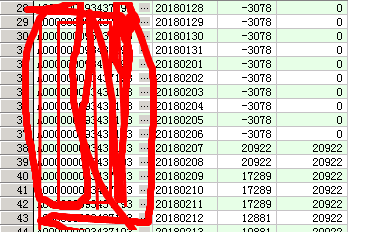THE END